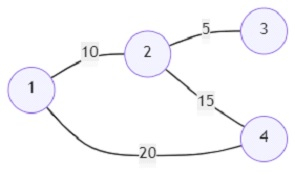# Program to find out the minimum cost path between the lowest value vertex to the highest value vertex (Python)

Suppose we are given an undirected, weighted graph and are asked to find out the path with the minimum possible travel cost from a particular node to another particular node. The travel cost is calculated as the following: suppose there is a path between vertex A to vertex C as A-> B-> C. The cost of travel from A to B is 10 and the cost of travel from B to C is 20. The cost of travel from A to C will be (cost of traveling from A to B) + (difference of traveling cost from B to C and the cumulative cost of traveling to node B). So that will translate to 10 + (20 - 10) = 20. We shall have to find out the minimum possible traveling values within the first node to the last node (the node with the lowest value to the node with the highest value) in a given graph.

So, if the input is likethen the output will be 15.

There exist two paths between vertices 1 and 4. The optimal path is 1->2->4, the cost of the path is 10 + (15 - 10) = 15. Otherwise, the path will cost 20.

To solve this, we will follow these steps −

• adjList := a new map containing empty lists
• for each item in edges, do
• u := item
• v := item
• w := item
• insert pair (w,v) at the end of adjList[u]
• insert pair (w,u) at the end of adjList[v]
• q := a new heap
• v_list := a new set
• insert (0,1) at the end of q
• flag := True
• while q is not empty, do
• c := pop smallest item from q
• if c is present in v_list, then
• go for next iteration
• if c is same as n, then
• flag := False
• return c
• for each u in adjList[c], do
• if u is not present in v_list, then
• out := maximum of (u, c ,u)
• push out into heap q
• if flag is True then
• return -1

## Example

Let us see the following implementation to get better understanding −

from collections import defaultdict
import heapq
def solve(n, edges):
for item in edges:
u, v, w = map(int, item)
q = []
v_list = set()
q.append((0,1))
flag = True
while q:
c = heapq.heappop(q)
if c in v_list:
continue
if c == n:
flag = False
return c
if u not in v_list:
out = (max(u,c),u)
heapq.heappush(q,out)
if flag:
return -1

print(solve(4, [(1, 2, 10), (2, 3, 5), (2, 4, 15), (1, 4, 20)]))


## Input

4, [(1, 2, 10), (2, 3, 5), (2, 4, 15), (1, 4, 20)]

## Output

15